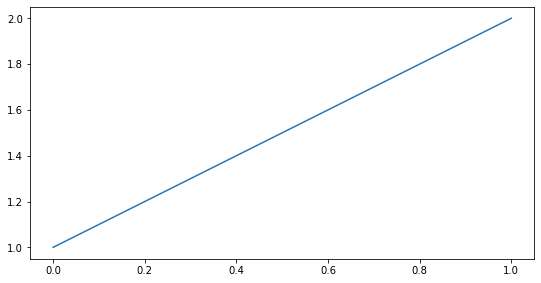# Save figure as file from iPython notebook using Matplotlib

To save a figure as a file from iPython, we can take the following steps−

• Create a new figure or activate an existing figure.
• Plot the given list.
• Save the plot using savefig() method.

## Example

from matplotlib import pyplot as plt
plt.rcParams["figure.figsize"] = [7.00, 3.50]
plt.rcParams["figure.autolayout"] = True
fig = plt.figure()
ax = fig.add_axes([1, 1, 1, 1])
plt.plot([1, 2])
plt.savefig('test.png', bbox_inches='tight')

## Output

When we execute the code, it will save the following plot as "test.png".